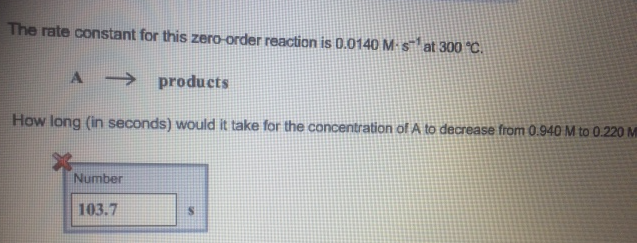# Problem: The rate constant for this zero order reaction is 0, 0140 M s^-1 at 300 degree C.A ----&gt; productsHow long (in seconds) would it take for the concentration of A to decrease from 0.940 M to 0.220 M

###### FREE Expert Solution
81% (281 ratings)###### Problem Details

The rate constant for this zero order reaction is 0, 0140 M s^-1 at 300 degree C.

A ----> products

How long (in seconds) would it take for the concentration of A to decrease from 0.940 M to 0.220 M